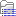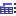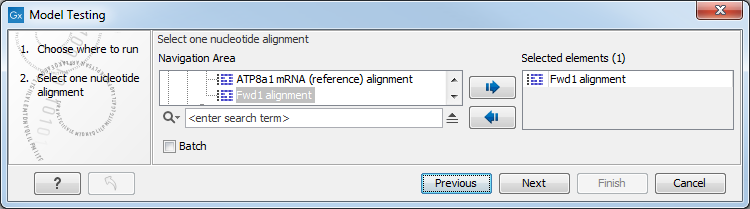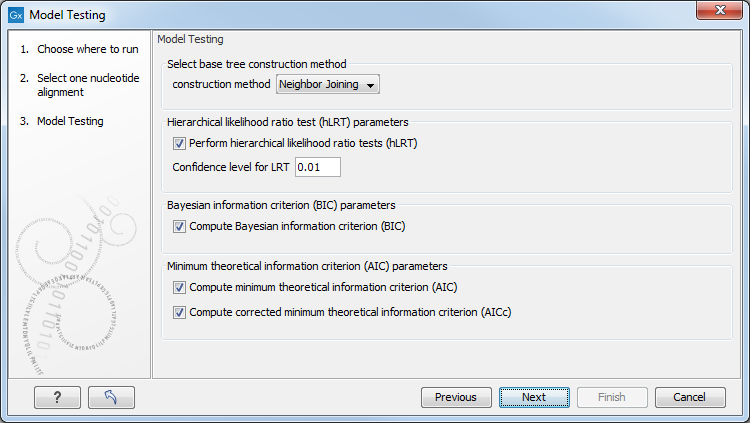# Model Testing

As the "Model Testing" tool can help identify the best substitution model (20.4.1) to be used for "Maximum Likelihood Phylogeny" tree construction, it is recommended to do "Model Testing" before running the "Maximum Likelihood Phylogeny" tool.

The "Model Testing" tool uses four different statistical analyses:

• Hierarchical likelihood ratio test (hLRT)
• Bayesian information criterion (BIC)
• Minimum theoretical information criterion (AIC)
• Minimum corrected theoretical information criterion (AICc)

to test the substitution models:

To do model testing:

Toolbox | Classical Sequence Analysis () | Alignments and Trees ()| Model Testing ()

Select the alignment that you wish to use for the tree construction (figure 20.5):Figure 20.5: Select alignment for model testing.

Specify the parameters to be used for model testing (figure 20.6):Figure 20.6: Specify parameters for model testing.

• Select base tree construction method

A base tree (a guiding tree) is required in order to be able to determine which model(s) would be the most appropriate to use to make the best possible phylogenetic tree from a specific alignment. The topology of the base tree is used in the hierarchical likelihood ratio test (hLRT), and the base tree is used as starting point for topology exploration in Bayesian information criterion (BIC), Akaike information criterion (or minimum theoretical information criterion) (AIC), and AICc (AIC with a correction for the sample size) ranking.

• Construction method A base tree is created automatically using one of two methods from the "Create Tree" tool:
• The UPGMA method. Assumes constant rate of evolution.
• The Neighbor Joining method. Well suited for trees with varying rates of evolution.

• Hierarchical likelihood ratio test (hLRT) parameters A statistical test of the goodness-of-fit between two models that compares a relatively more complex model to a simpler model to see if it fits a particular dataset significantly better.
• Perform hierarchical likelihood ratio test (hLRT)
• Confidence level for LRT The confidence level used in the likelihood ratio tests.
• Bayesian information criterion (BIC) parameters
• Compute Bayesian information criterion (BIC) Rank substitution models based on Bayesian information criterion (BIC). Formula used is BIC = -2ln(L)+Kln(n), where ln(L) is the log-likelihood of the best tree, K is the number of parameters in the model, and ln(n) is the logarithm of the length of the alignment.
• Minimum theoretical information criterion (AIC) parameters
• Compute minimum theoretical information criterion (AIC) Rank substitution models based on minimum theoretical information criterion (AIC). Formula used is AIC = -2ln(L)+2K, where ln(L) is the log-likelihood of the best tree, K is the number of parameters in the model.
• Compute corrected minimum theoretical information criterion (AIC) Rank substitution models based on minimum corrected theoretical information criterion (AICc). Formula used is AICc = -2ln(L)+2K+2K(K+1)/(n-K-1), where ln(L) is the log-likelihood of the best tree, K is the number of parameters in the model, n is the length of the alignment. AICc is recommended over AIC roughly when n/K is less than 40.

The output from model testing is a report that lists all test results in table format. For each tested model the report indicate whether it is recommended to use rate variation or not. Topology variation is recommended in all cases.

From the listed test results, it is up to the user to select the most appropriate model. The different statistical tests will usually agree on which models to recommend although variations may occur. Hence, in order to select the best possible model, it is recommended to select the model that has proven to be the best by most tests.